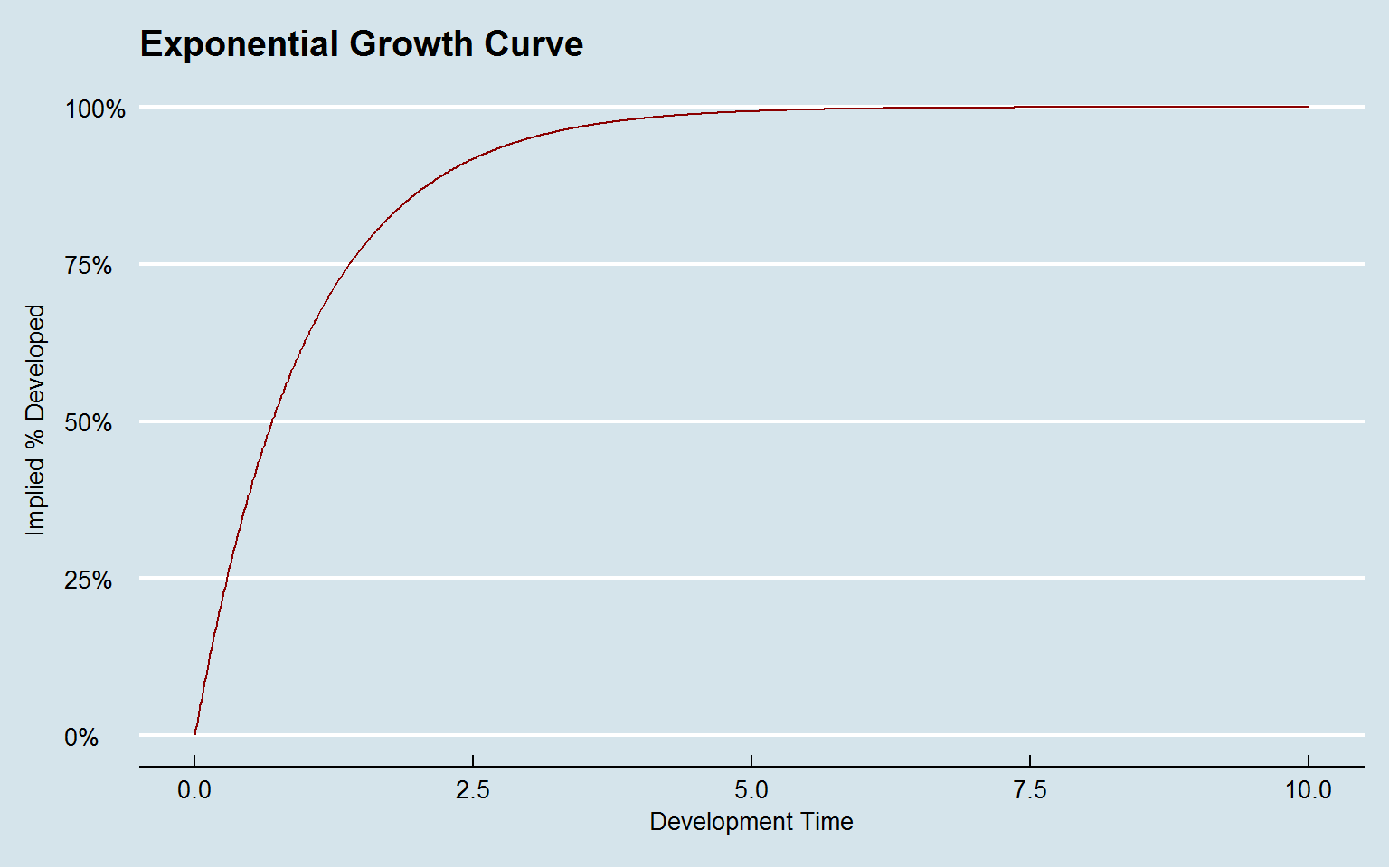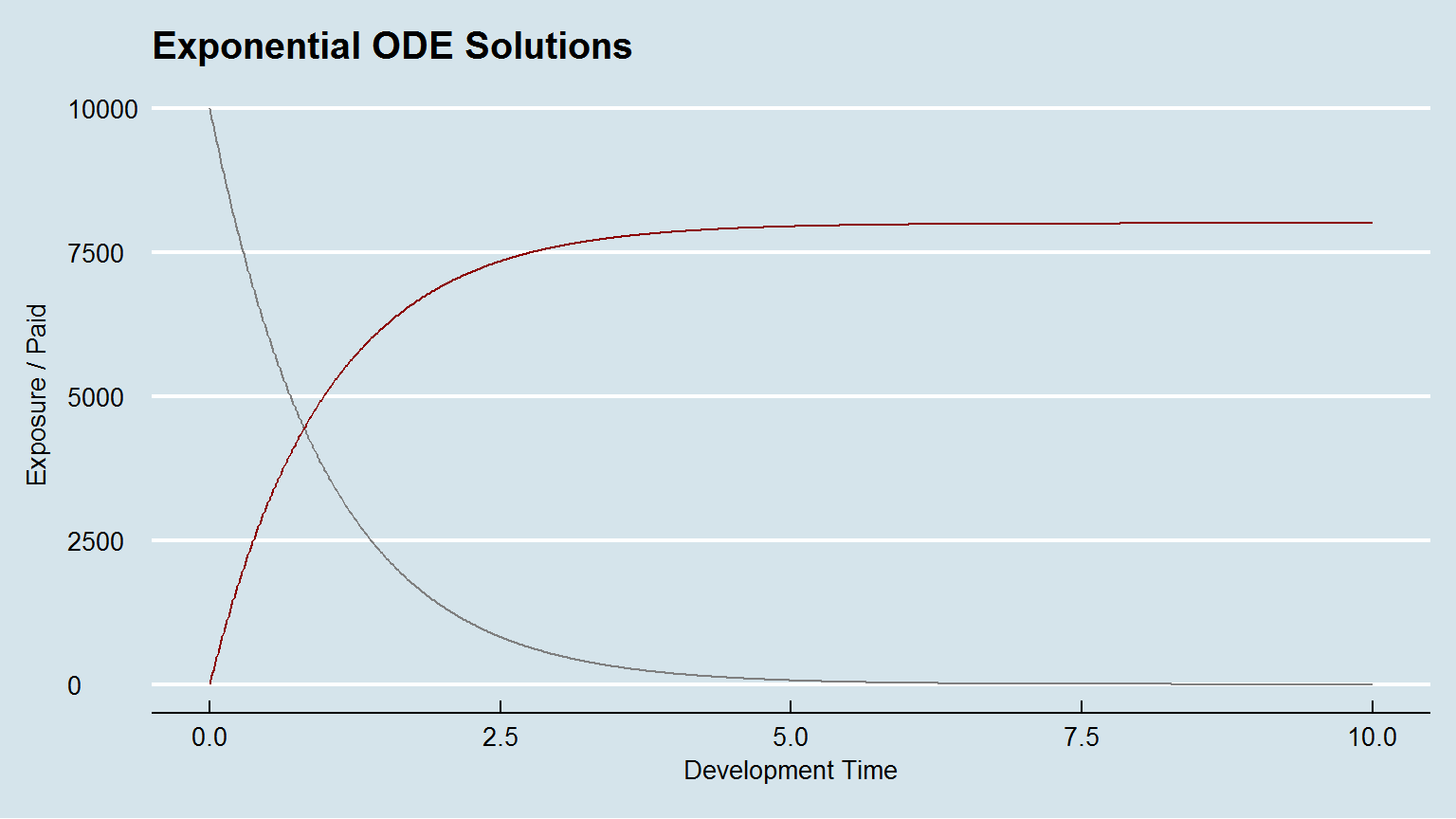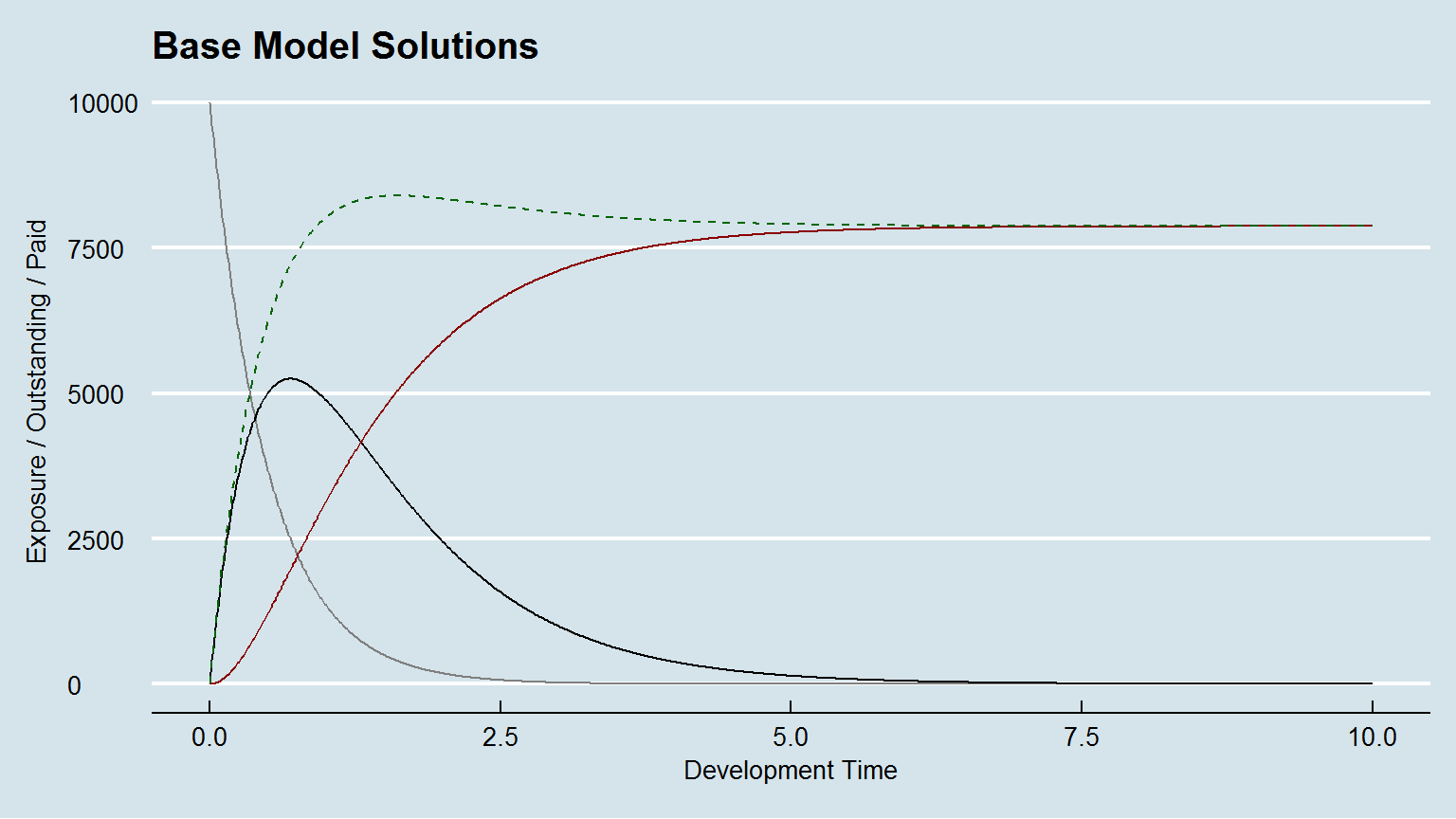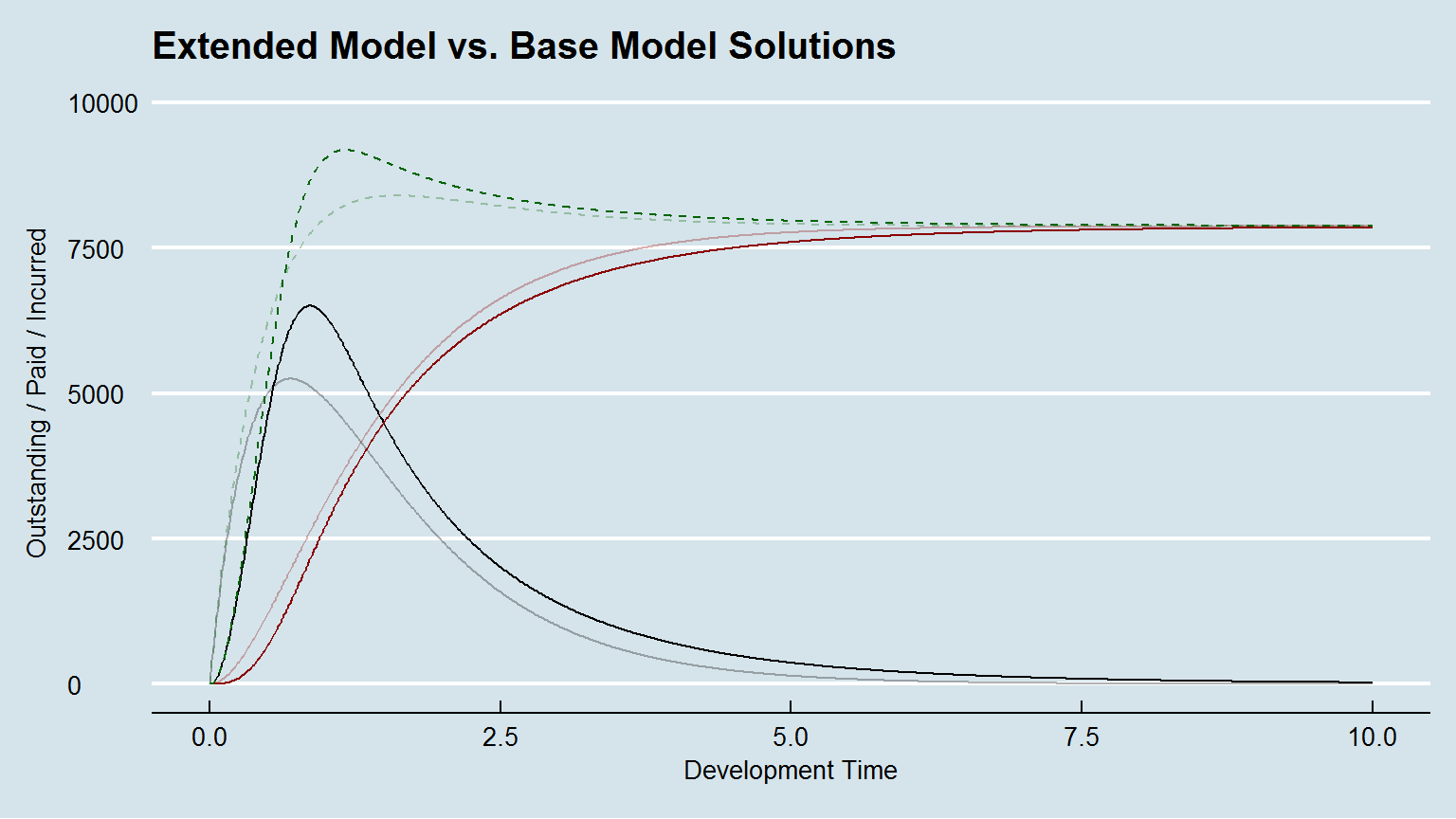# Extending the Growth Curve Approach

## Exponential Growth Function

• Exponential growth for rate of payment $$k_{p}$$ defined as: $GF_{t} = 1-e^{-k_{p} \cdot t}$
•## Exponential Growth as an ODE system

• Define $$EX_{t}$$ as exposure % at development time t

• Assume $$EX_{0} = 1$$ and $$EX_{t} + GF_{t} = 1$$

• Growth function is a solution to ODEs:

• $dEX_{t}/dt = -k_{p} \cdot EX_{t}$ $dGF_{t}/dt = k_{p} \cdot EX_{t}$

• $EX_{t} = e^{-k_{p} \cdot t}$ $GF_{t} = 1-e^{-k_{p} \cdot t}$

## Exponential Growth Model as an ODE system

• Assume $$EX_{0} = \text{Premiums} = P$$

• Define $$PD_{t}$$ as cumulative paid claims at time t

• Allow a multiple of exposure, $$ULR$$, to become paid

• $dEX_{t}/dt = -k_{p} \cdot EX_{t}$ $dPD_{t}/dt = k_{p} \cdot ULR \cdot EX_{t}$
• $EX_{t} = P \cdot (1-GF_{t})$ $PD_{t} = P \cdot ULR \cdot GF_{t}$## Compartmental Model Representation

• Systems of ODEs can be written schematically as compartmental models:
• Readily extensible framework for modelling the claims process

# Compartmental Reserving Models

## Base Model Schematic

• Introduce additional compartment for reported claims
• Define $$k_{er}$$ as rate claims occur and are reported

• Split $$ULR$$ into multiplicative components:
• Reported Loss Ratio ($$RLR$$)
• Reserve Robustness Factor ($$RRF$$)

## Base Model ODEs

• \begin{gathered} \begin{align} \tfrac{dEX_{t}}{dt} &= -k_{er} \cdot EX_{t} \\ \tfrac{dOS_{t}}{dt} &= k_{er} \cdot RLR \cdot EX_{t} - k_{p} \cdot OS_{t} \\ \tfrac{dPD_{t}}{dt} &= k_{p} \cdot RRF \cdot OS_{t} \end{align} \end{gathered}
•## Extending the Model

• Constant rates of reporting and payment rarely hold
• Delays in reporting post-incident
• Payment delays for larger claims

• Allow $$k_{er}$$ and $$k_{p}$$ to depend on development time, $$t$$
• Many potential functions

• $k_{er}(t) = \beta_{er} \cdot t$ $k_{p}(t) = \beta_{1,p} / (\beta_{2,p} + t)$

## Extended Model ODEs

• \begin{gathered} \begin{align} \tfrac{dEX_{t}}{dt} &= -\beta_{er} \cdot t \cdot EX_{t} \\ \tfrac{dOS_{t}}{dt} &= \beta_{er} \cdot t \cdot RLR \cdot EX_{t} - \tfrac{\beta_{1,p}} {(\beta_{2,p} + t)} \cdot OS_{t} \\ \tfrac{dPD_{t}}{dt} &= \tfrac{\beta_{1,p}} {(\beta_{2,p} + t)} \cdot RRF \cdot OS_{t} \end{align} \end{gathered}
•# Hierarchical Compartmental Reserving Model

## Model Specification

• \begin{aligned} \begin{bmatrix} OS_{ij}, PD_{ij} \end{bmatrix}^{T} = \ y_{ij} &\thicksim \mathcal{N}(\mu_{ij},\ \sigma^{2}_{[\delta]}) \\ \mu_{ij} &= P_{i} \cdot f(\delta_{ij}, t_{j}, k_{er}, RLR_{[i]}, k_{p}, RRF_{[i]}) \\ \\ f(\delta_{ij},t_{j},\dots) & = (1 - \delta_{ij}) \cdot \hat {\mbox{OS}}(t_{j}, k_{er}, RLR_{[i]}, k_{p}) \ + \\ & \ \ \ \ \ \ \delta_{ij} \cdot \hat {\mbox{PD}}( t_{j}, k_{er}, RLR_{[i]}, k_{p}, RRF_{[i]}) \\ \\ \delta_{ij} &= \begin{cases} 0 & \text{if } y_{ij} = OS_{ij} \\ 1 & \text{if } y_{ij} = PD_{ij} \end{cases} \\ \\ \begin{pmatrix} RLR_{[i]} \\ RRF_{[i]}\end{pmatrix} &\thicksim \mathcal{N} \left( \begin{pmatrix} \mu_{RLR} \\ \mu_{RRF} \end{pmatrix}, \begin{pmatrix} \sigma_{_{RLR}}^2 & 0\\ 0 & \sigma_{_{RRF}}^2 \end{pmatrix} \right) \end{aligned}

## Priors

• Prior assumptions driven by domain knowledge:
• Profitability
• Case reserving strength
• Reporting / Payment speed

## ‘brms’ Code

fml <-
(1 - delta) * (RLR*ker/(ker - kp) * (exp(-kp*dev) - exp(-ker*dev))) +
delta * (RLR*RRF/(ker - kp) * (ker *(1 - exp(-kp*dev)) - kp*(1 - exp(-ker*dev))))
)

b1 <- brm(bf(fml,
ker ~ 1, kp ~ 1,
RLR ~ 1 + (1 | accident_year),
RRF ~ 1 + (1 | accident_year),
sigma ~ 0 + deltaf,
nl = TRUE),
data = lossData0[cal <= max(accident_year)],
family = brmsfamily("gaussian", link_sigma = "log"),
prior = c(prior(gamma(4, 5), nlpar = "RLR", lb=0),
prior(gamma(4, 5), nlpar = "RRF", lb=0),
prior(gamma(3, 2), nlpar = "ker", lb=0),
prior(gamma(3, 4), nlpar = "kp", lb=0)),
control = list(adapt_delta = 0.999, max_treedepth=15),
seed = 1234, iter = 1000)

## Exercise Questions

• Is a Normal observation model appropriate?

• How sensitive is the model to alternative priors?

• Are $$RLR_{i}$$ and $$RRF_{i}$$ likely to be independent?

• How might we increase model flexibility?